## Figures index

#### Nasib Al Habib, Shuva Ghosh

American Journal of Industrial Engineering. 2018, 5(1), 1-11 doi:10.12691/ajie-5-1-1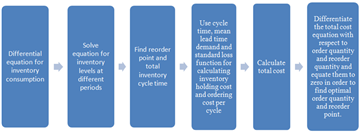• Figure 1. Sequential steps in model formulation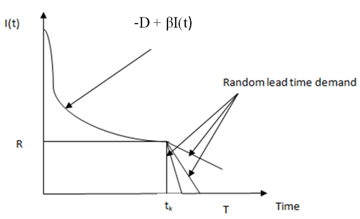• Figure 2. Graphical representation of the inventory system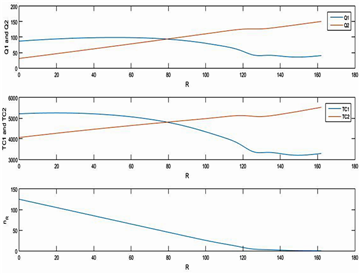• Figure 3. Optimal values of R, Q, Total Cost and shortage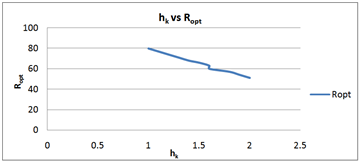• Figure 4. Effects of holding cost on reorder quantity• Figure 5. Effect of holding Cost on quantity ordered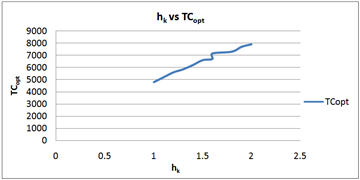• Figure 6. Effect of holding Cost on total Cost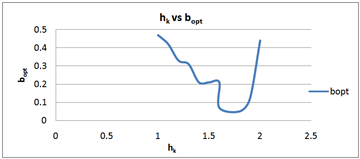• Figure 7. Effect of holding Cost on fill-rate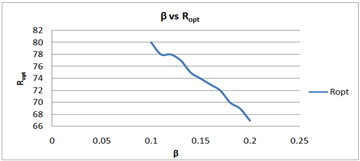• Figure 8. Effect of demand elasticity on reorder quantity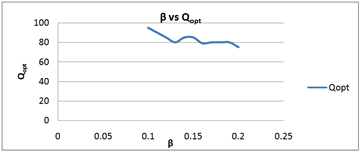• Figure 9. Effect of demand elasticity on order quantity• Figure 10. Effect of demand elasticity on total Cost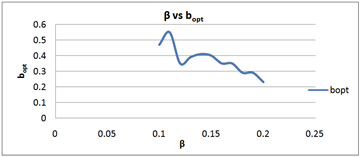• Figure 11. Effect of demand elasticity on fill-rate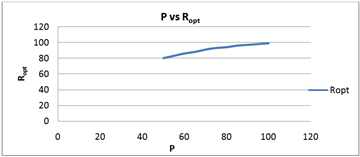• Figure 12. Effect of shortage cost on reorder quantity• Figure 13. Effect of shortage cost on order quantity Q• Figure 14. Effect of shortage cost on total cost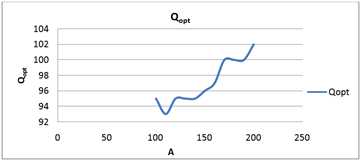• Figure 16. Effect of ordering cost on order quantity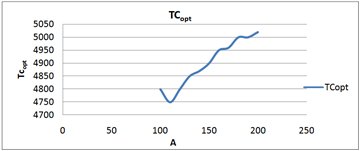• Figure 17. Effect of ordering cost on total cost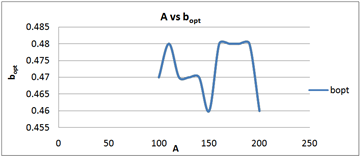• Figure 18. Effect of ordering cost on fill-rate